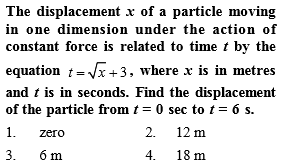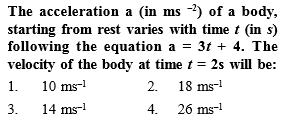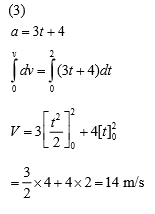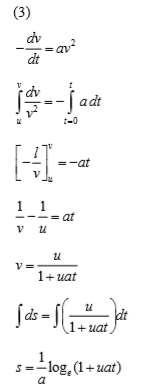Concept Videos :-

#42 Non Uniform Acceleration
#43 Solved Examples 29
#44 Solved Examples 30

Concept Questions :-

Non-uniform acceleration

(1)

Difficulty Level:

• 60%
• 13%
• 14%
• 15%Concept Videos :-

#42 Non Uniform Acceleration
#43 Solved Examples 29
#44 Solved Examples 30

Concept Questions :-

Non-uniform accelerationDifficulty Level:

• 22%
• 11%
• 65%
• 3%

A point moves in a straight line under the retardation a${\mathrm{v}}^{2}$. If the initial velocity is u, the distance covered in 't' seconds is-

1.  $\mathrm{aut}$

2.

3.

4.

Concept Videos :-

#42 Non Uniform Acceleration
#43 Solved Examples 29
#44 Solved Examples 30

Concept Questions :-

Non-uniform accelerationDifficulty Level:

• 16%
• 24%
• 56%
• 6%

A body starts from the origin and moves along the X-axis such that the velocity at any instant is given by $\left(4{t}^{3}-2t\right)$, where t is in sec and velocity in m/s. What is the acceleration of the particle, when it is 2 m from the origin ?

(1) 28 m/s2

(2) 22 m/s2

(3) 12 m/s2

(4) 10 m/s2

Concept Videos :-

#42 Non Uniform Acceleration
#43 Solved Examples 29
#44 Solved Examples 30

Concept Questions :-

Non-uniform acceleration

(2) $v=4{t}^{3}-2t$(given) ∴ $a=\frac{dv}{dt}=12{t}^{2}-2$

and $x={\int }_{0}^{t}v\text{\hspace{0.17em}}dt={\int }_{0}^{t}\left(4{t}^{3}-2t\right)\text{\hspace{0.17em}}dt={t}^{4}-{t}^{2}$

When particle is at 2m from the origin ${t}^{4}-{t}^{2}=2$

${t}^{4}-{t}^{2}-2=0$$\left({t}^{2}-2\right)\text{\hspace{0.17em}}\left({t}^{2}+1\right)=0$$t=\sqrt{2}\text{\hspace{0.17em}}\mathrm{sec}$

Acceleration at $t=\sqrt{2}\text{\hspace{0.17em}}\mathrm{sec}$ given by,

$a=12{t}^{2}-2=12×2-2$ = $22\text{\hspace{0.17em}}m/{s}^{2}$

Difficulty Level:

• 13%
• 67%
• 14%
• 9%

The initial velocity of a particle is u (at t = 0) and the acceleration f is given by at. Which of the following relation is valid

(1) $v=u+a{t}^{2}$

(2) $v=u+a\frac{{t}^{2}}{2}$

(3) $v=u+at$

(4) v = u

Concept Videos :-

#42 Non Uniform Acceleration
#43 Solved Examples 29
#44 Solved Examples 30

Concept Questions :-

Non-uniform acceleration

(2) If acceleration is variable (depends on time) then

$v=u+\frac{a{t}^{2}}{2}$

Difficulty Level:

• 27%
• 26%
• 31%
• 19%

The velocity of a body depends on time according to the equation $v=20+0.1{t}^{2}$. The body is undergoing

(1) Uniform acceleration

(2) Uniform retardation

(3) Non-uniform acceleration

(4) Zero acceleration

Concept Videos :-

#42 Non Uniform Acceleration
#43 Solved Examples 29
#44 Solved Examples 30

Concept Questions :-

Non-uniform acceleration

(3) Acceleration $a=\frac{dv}{dt}=0.1×2t=0.2t$

Which is time dependent i.e. non-uniform acceleration.

Difficulty Level:

• 30%
• 8%
• 60%
• 5%

A rocket is fired upward from the earth's surface such that it creates an acceleration of 19.6 m/sec2. If after 5 sec its engine is switched off, the maximum height of the rocket from earth's surface would be [MP PET 1995]

(1) 245 m

(2) 490 m

(3) 980 m

(4) 735 m

Concept Videos :-

#42 Non Uniform Acceleration
#43 Solved Examples 29
#44 Solved Examples 30

Concept Questions :-

Non-uniform acceleration

(4) Given $a=19.6\text{\hspace{0.17em}}m/{s}^{2}=2g$

Resultant velocity of the rocket after 5 sec

$v=2g×5=10g\text{\hspace{0.17em}}m/s$

Height achieved after 5 sec, ${h}_{1}=\frac{1}{2}×2g×25=245m$

On switching off the engine it goes up to height ${h}_{2}$ where its velocity becomes zero.

$0={\left(10g\right)}^{2}-2g{h}_{2}⇒{h}_{2}=490m$

∴ Total height of rocket $=245+490=735\text{\hspace{0.17em}}m$

Difficulty Level:

• 29%
• 22%
• 8%
• 42%

A balloon starts rising from the ground with an acceleration of 1.25 m/s2 . After 8s, a stone is released from the balloon. The stone will (g = 10 m/s2

(1) Reach the ground in 4 second

(2) Begin to move down after being released

(3) Have a displacement of 50 m

(4) Cover a distance of 40 m in reaching the ground

Concept Videos :-

#42 Non Uniform Acceleration
#43 Solved Examples 29
#44 Solved Examples 30

Concept Questions :-

Non-uniform acceleration

(1)

When the stone is released from the balloon. Its height $h=\frac{1}{2}a{t}^{2}=\frac{1}{2}×1.25×{\left(8\right)}^{2}=40\text{\hspace{0.17em}}m$ and velocity

$v=at=1.25×8=10\text{\hspace{0.17em}}m/s$

Difficulty Level:

• 50%
• 18%
• 15%
• 19%

The acceleration of a particle is increasing linearly with time t as bt. The particle starts from the origin with an initial velocity v0 The distance travelled by the particle in time t will be

(1) ${v}_{0}t+\frac{1}{3}b{t}^{2}$

(2) ${v}_{0}t+\frac{1}{3}b{t}^{3}$

(3) ${v}_{0}t+\frac{1}{6}b{t}^{3}$

(4) ${v}_{0}t+\frac{1}{2}b{t}^{2}$

Concept Videos :-

#42 Non Uniform Acceleration
#43 Solved Examples 29
#44 Solved Examples 30

Concept Questions :-

Non-uniform acceleration

(3) $\frac{dv}{dt}=bt⇒dv=bt\text{\hspace{0.17em}}dt⇒v=\frac{b{t}^{2}}{2}+{K}_{1}$

At $t=0,\text{\hspace{0.17em}}v={v}_{0}⇒{K}_{1}={v}_{0}$

We get $v=\frac{1}{2}b{t}^{2}+{v}_{0}$

Again $\frac{dx}{dt}=\frac{1}{2}b{t}^{2}+{v}_{0}⇒x=\frac{1}{2}\frac{b{t}^{2}}{3}+{v}_{0}t+{K}_{2}$

At $t=0,\text{\hspace{0.17em}}x=0⇒{K}_{2}=0$

Difficulty Level:

• 6%
• 21%
• 49%
• 26%

The acceleration of a particle starting from rest, varies with time according to the relation A = – 2 sinω t. The displacement of this particle at a time t will be

(1) $-\frac{1}{2}\text{\hspace{0.17em}}\left(a{\omega }^{2}\mathrm{sin}\omega \text{\hspace{0.17em}}t\right)\text{\hspace{0.17em}}{t}^{2}$

(2) $a\omega \text{\hspace{0.17em}}\mathrm{sin}\omega \text{\hspace{0.17em}}t$

(3) $a\omega \text{\hspace{0.17em}}\mathrm{cos}\omega \text{\hspace{0.17em}}t$

(4) $a\text{\hspace{0.17em}}\mathrm{sin}\omega \text{\hspace{0.17em}}t$

Concept Videos :-

#42 Non Uniform Acceleration
#43 Solved Examples 29
#44 Solved Examples 30

Concept Questions :-

Non-uniform acceleration

(4) Velocity $v=\int A\text{\hspace{0.17em}}dt=\int \left(-a{\omega }^{2}\mathrm{sin}\omega t\right)\text{\hspace{0.17em}}dt=a\omega \mathrm{cos}\omega t$

Displacement x $=\int v\text{\hspace{0.17em}}dt=\int a\omega \mathrm{cos}\omega t\text{\hspace{0.17em}}dt=a\mathrm{sin}\omega t$

Difficulty Level:

• 20%
• 17%
• 14%
• 50%